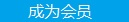您可以捐助，支持我们的公益事业。 1元 10元 50元 认证码：必填求知 文章 文库 Lib 视频 Code iProcess 课程 角色 咨询 工具 火云堂 讲座吧 成长之路要资料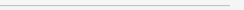订阅捐助
C++静态库与动态库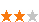1786 次浏览     评价： 好 中 差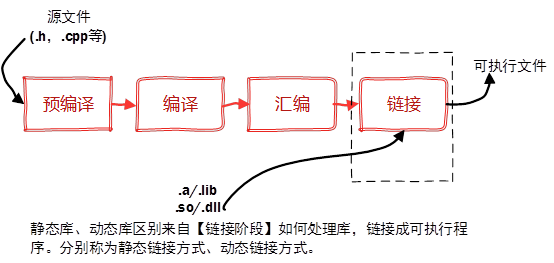StaticMath.h头文件

 `#pragma once` class StaticMath { public: StaticMath(void); ~StaticMath(void); static double add(double a, double b);//加法 static double sub(double a, double b);//减法 static double mul(double a, double b);//乘法 static double div(double a, double b);//除法 void print(); };

Linux下使用ar工具、Windows下vs使用lib.exe，将目标文件压缩到一起，并且对其进行编号和索引，以便于查找和检索。一般创建静态库的步骤如图所示：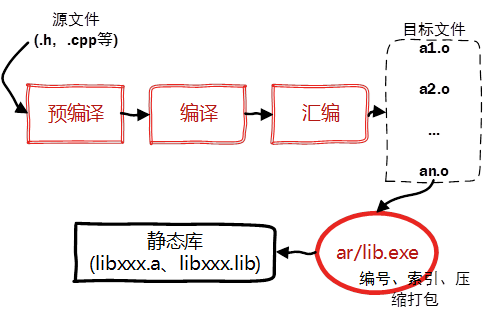Linux下创建与使用静态库

Linux静态库命名规则

Linux静态库命名规范，必须是"lib[your_library_name].a"：lib为前缀，中间是静态库名，扩展名为.a。

 `g++ -c StaticMath.cpp`

 `ar -crv libstaticmath.a StaticMath.o`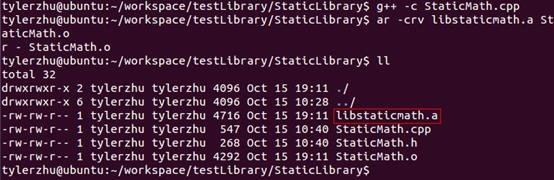`#include "StaticMath.h"` #include using namespace std; int main(int argc, char* argv[]) { double a = 10; double b = 2; cout << "a + b = " << StaticMath::add(a, b) << endl; cout << "a - b = " << StaticMath::sub(a, b) << endl; cout << "a * b = " << StaticMath::mul(a, b) << endl; cout << "a / b = " << StaticMath::div(a, b) << endl; StaticMath sm; sm.print(); system("pause"); return 0; }

Linux下使用静态库，只需要在编译的时候，指定静态库的搜索路径（-L选项）、指定静态库名（不需要lib前缀和.a后缀，-l选项）。

 `# g++ TestStaticLibrary.cpp -L../StaticLibrary -lstaticmath`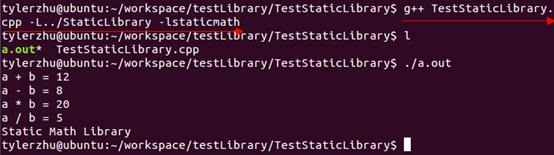-L：表示要连接的库所在目录

-l：指定链接时需要的动态库，编译器查找动态连接库时有隐含的命名规则，即在给出的名字前面加上lib，后面加上.a或.so来确定库的名称。

Windows下创建与使用静态库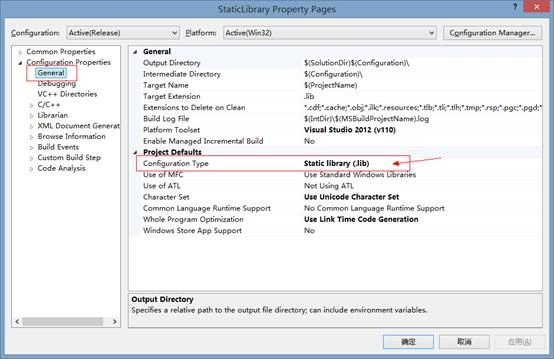Build项目即可生成静态库。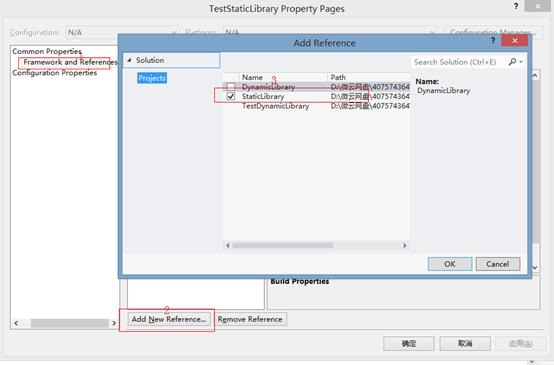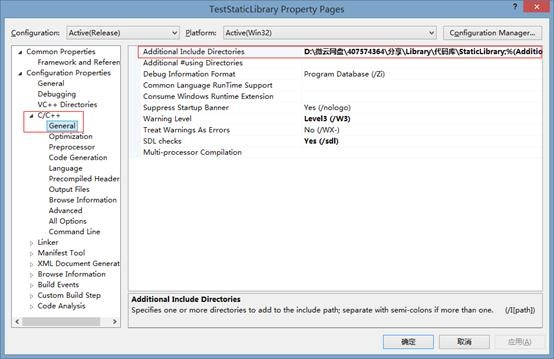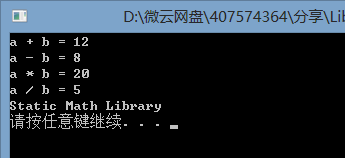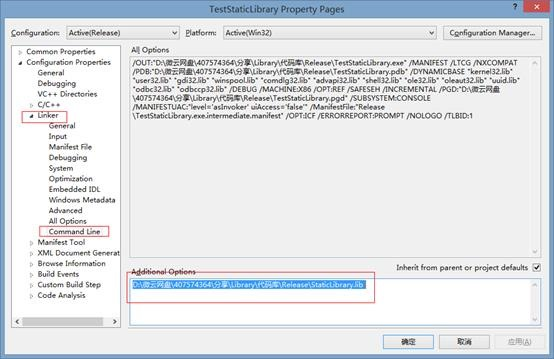“属性面板”è”配置属性”è “链接器”è”常规”，附加依赖库目录中输入，静态库所在目录；

“属性面板”è”配置属性”è “链接器”è”输入”，附加依赖库中输入静态库名StaticLibrary.lib。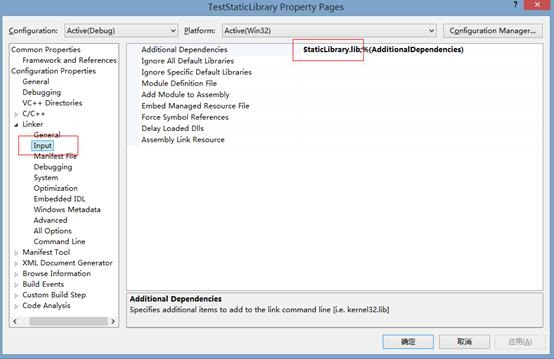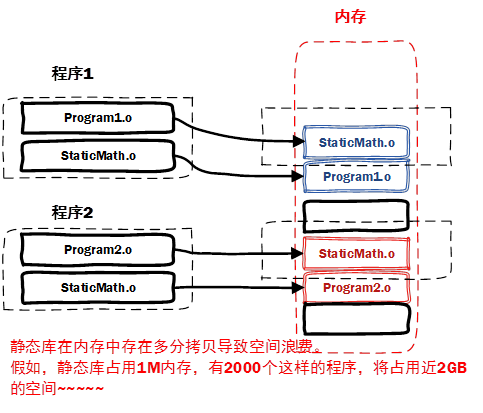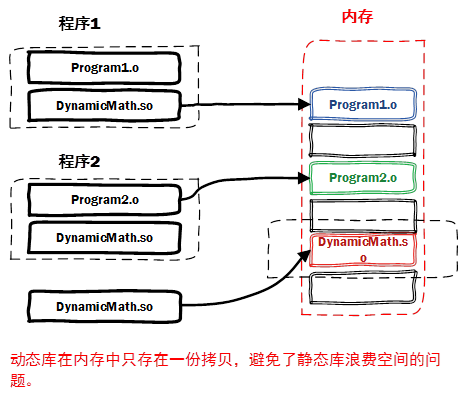Window与Linux执行文件格式不同，在创建动态库的时候有一些差异。

Linux下gcc编译的执行文件默认是ELF格式，不需要初始化入口，亦不需要函数做特别的声明，编写比较方便。

Linux下创建与使用动态库

linux动态库的命名规则

DynamicMath.h头文件

 `#pragma once` class DynamicMath { public: DynamicMath(void); ~DynamicMath(void); static double add(double a, double b);//?ó・¨ static double sub(double a, double b);//??・¨ static double mul(double a, double b);//3?・¨ static double div(double a, double b);//3y・¨ void print(); };

 `g++ -fPIC -c DynamicMath.cpp`

-fPIC 创建与地址无关的编译程序（pic，position independent code），是为了能够在多个应用程序间共享。

 `g++ -shared -o libdynmath.so DynamicMath.o`

-shared指定生成动态链接库。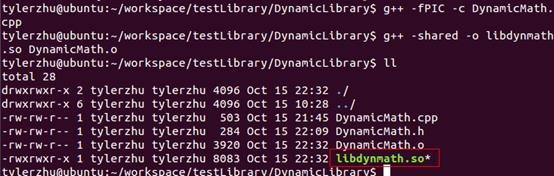`g++ -fPIC -shared -o libdynmath.so DynamicMath.cpp`

 `#include "../DynamicLibrary/DynamicMath.h"` #include using namespace std; int main(int argc, char* argv[]) { double a = 10; double b = 2; cout << "a + b = " << DynamicMath::add(a, b) << endl; cout << "a - b = " << DynamicMath::sub(a, b) << endl; cout << "a * b = " << DynamicMath::mul(a, b) << endl; cout << "a / b = " << DynamicMath::div(a, b) << endl; DynamicMath dyn; dyn.print(); return 0; }

 `g++ TestDynamicLibrary.cpp -L../DynamicLibrary -ldynmath`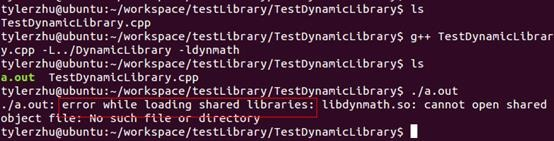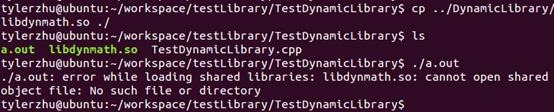2) 对于elf格式的可执行程序，是由ld-linux.so*来完成的，它先后搜索elf文件的 DT_RPATH段―环境变量LD_LIBRARY_PATH―/etc/ld.so.cache文件列表―/lib/,/usr/lib 目录找到库文件后将其载入内存。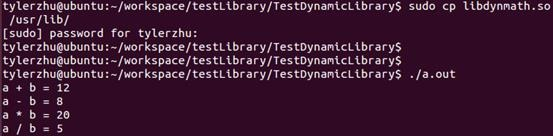Windows下创建与使用动态库

dllmain.cpp入口文件

 `// dllmain.cpp : Defines the entry point for the DLL application.` #include "stdafx.h" BOOL APIENTRY DllMain( HMODULE hModule, DWORD ul_reason_for_call, LPVOID lpReserved ) { switch (ul_reason_for_call) { case DLL_PROCESS_ATTACH: case DLL_THREAD_ATTACH: case DLL_THREAD_DETACH: case DLL_PROCESS_DETACH: break; } return TRUE; }

DynamicMath.h头文件

 `#pragma once` class DynamicMath { public: __declspec(dllexport) DynamicMath(void); __declspec(dllexport) ~DynamicMath(void); static __declspec(dllexport) double add(double a, double b);//加法 static __declspec(dllexport) double sub(double a, double b);//减法 static __declspec(dllexport) double mul(double a, double b);//乘法 static __declspec(dllexport) double div(double a, double b);//除法 __declspec(dllexport) void print(); };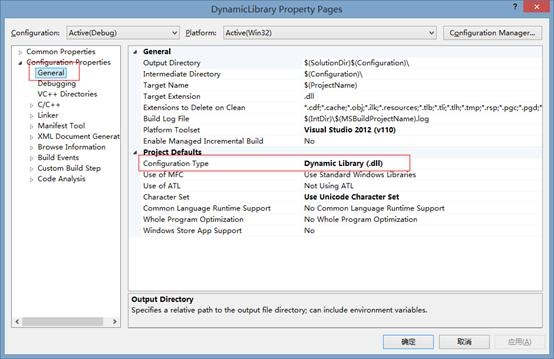Build项目即可生成动态库。

TestDynamicLibrary.cpp测试程序

 `#include "stdafx.h"` #include "DynamicMath.h" #include using namespace std; int _tmain(int argc, _TCHAR* argv[]) { double a = 10; double b = 2; cout << "a + b = " << DynamicMath::add(a, b) << endl; cout << "a - b = " << DynamicMath::sub(a, b) << endl; cout << "a * b = " << DynamicMath::mul(a, b) << endl; cout << "a / b = " << DynamicMath::div(a, b) << endl; DynamicMath dyn; dyn.print(); system("pause"); return 0; }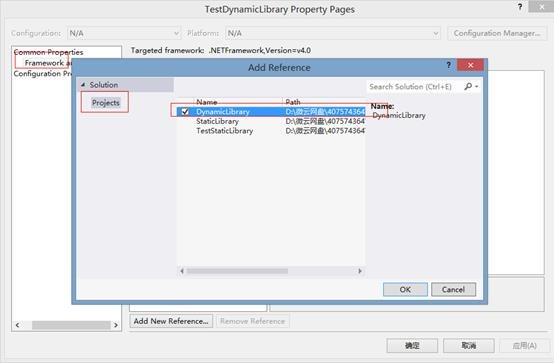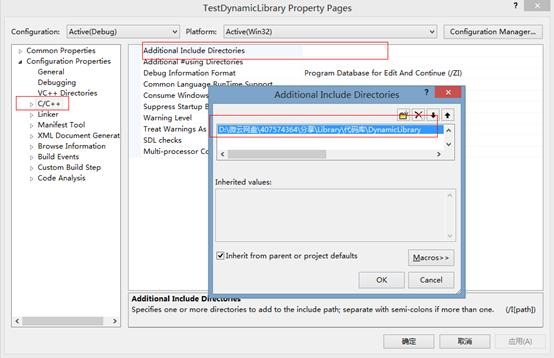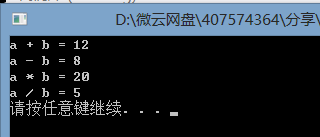“属性面板”è”配置属性”è “链接器”è”常规”，附加依赖库目录中输入，动态库所在目录；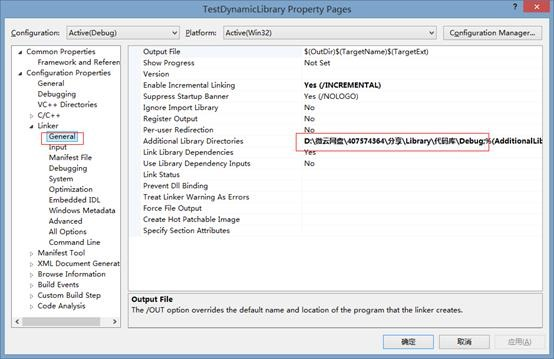“属性面板”è”配置属性”è “链接器”è”输入”，附加依赖库中输入动态库编译出来的DynamicLibrary.lib。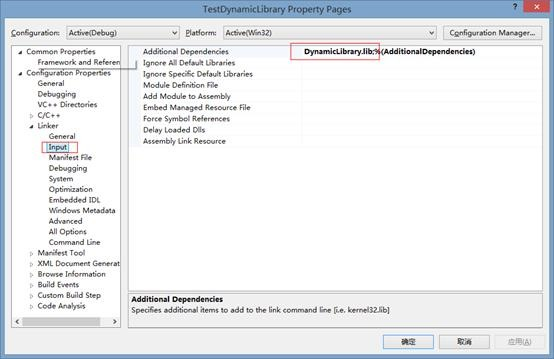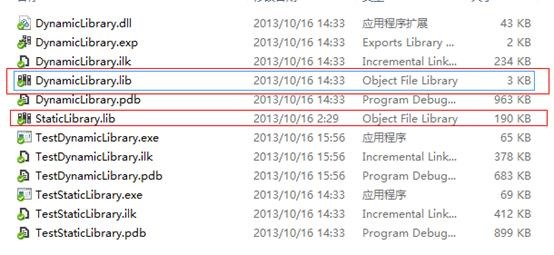StaticLibrary.lib的大小为190KB，DynamicLibrary.lib的大小为3KB，静态库对应的lib文件叫静态库，动态库对应的lib文件叫【导入库】。实际上静态库本身就包含了实际执行代码、符号表等等，而对于导入库而言，其实际的执行代码位于动态库中，导入库只包含了地址符号表等，确保程序找到对应函数的一些基本地址信息。

#include <dlfcn.h>，提供了下面几个接口：

void * dlopen( const char * pathname, int mode )：函数以指定模式打开指定的动态连接库文件，并返回一个句柄给调用进程。

void* dlsym(void* handle,const char* symbol)：dlsym根据动态链接库操作句柄(pHandle)与符号(symbol)，返回符号对应的地址。使用这个函数不但可以获取函数地址，也可以获取变量地址。

int dlclose (void *handle)：dlclose用于关闭指定句柄的动态链接库，只有当此动态链接库的使用计数为0时,才会真正被系统卸载。

const char *dlerror(void)：当动态链接库操作函数执行失败时，dlerror可以返回出错信息，返回值为NULL时表示操作函数执行成功。

name mangling可以通过extern "C"解决。C++有个特定的关键字用来声明采用C binding的函数：extern "C" 。用 extern "C"声明的函数将使用函数名作符号名，就像C函数一样。因此，只有非成员函数才能被声明为extern "C"，并且不能被重载。尽管限制多多，extern "C"函数还是非常有用，因为它们可以象C函数一样被dlopen动态加载。冠以extern "C"限定符后，并不意味着函数中无法使用C++代码了，相反，它仍然是一个完全的C++函数，可以使用任何C++特性和各种类型的参数。

“显式”使用C++动态库中的Class是非常繁琐和危险的事情，因此能用“隐式”就不要用“显式”，能静态就不要用动态。1786 次浏览  评价: 好 中 差订阅捐助

 深度解析：清理烂代码 如何编写出拥抱变化的代码 重构-使代码更简洁优美 团队项目开发"编码规范"系列文章
 重构-改善既有代码的设计 软件重构v2 代码整洁之道 高质量编程规范
 基于HTML5客户端、Web端的应用开发 HTML 5+CSS 开发 嵌入式C高质量编程 C++高级编程

 Visual C++编程命名规则 任何时候都适用的20个C++技巧 C语言进阶 串口驱动分析 轻轻松松从C一路走到C++ C++编程思想

 C++并发处理+单元测试 C++程序开发 C++高级编程 C/C++开发 C++设计模式 C/C++单元测试

 北京 嵌入式C高质量编程 中国航空 嵌入式C高质量编程 华为 C++高级编程 北京 C++高级编程 丹佛斯 C++高级编程 北大方正 C语言单元测试 罗克韦尔 C++单元测试每天2个文档/视频 扫描微信二维码订阅
 订阅技术月刊 获得每月300个技术资源